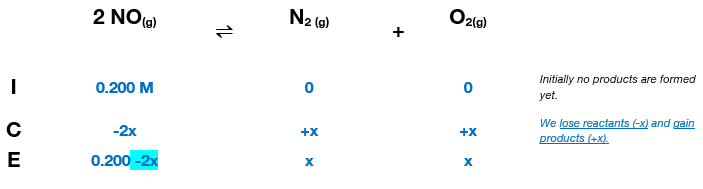# Problem: At 2000°C the equilibrium constant for the reaction2NO(g) ⇌ N2(g) + O2(g)is Kc = 2.4 × 103. The initial concentration of NO is 0.200 M .a) What is the equilibrium concentration of NO? b) What is the equilibrium concentration of N2? c) What is the equilibrium concentration of O2?

###### FREE Expert Solution

Construct an ICE chart to detemrinet the equilibrium concentration.$\overline{){{\mathbf{K}}}_{{\mathbf{c}}}{\mathbf{=}}\frac{\mathbf{products}}{\mathbf{reactants}}{\mathbf{=}}\frac{\left[{N}_{2}\right]\left[{O}_{2}\right]}{{\left[\mathrm{NO}\right]}^{\mathbf{2}}}}$

${\mathbf{2}}{\mathbf{.}}{\mathbf{4}}{\mathbf{×}}{{\mathbf{10}}}^{{\mathbf{3}}}{\mathbf{=}}\frac{\left(x\right)\left(x\right)}{\mathbf{0}\mathbf{.}\mathbf{200}\mathbf{-}\mathbf{2}\mathbf{x}}$

$\left[2.4×{10}^{3}=\frac{{x}^{2}}{\overline{)0.200-2x}}\right]\overline{)\left[{0}{.}{200}{-}{2}{x}\right]}$

$\mathbf{\left(}\mathbf{2}\mathbf{.}\mathbf{4}\mathbf{×}{\mathbf{10}}^{\mathbf{3}}\mathbf{\right)}\mathbf{\left(}\mathbf{0}\mathbf{.}\mathbf{200}\mathbf{-}\mathbf{2}\mathbf{x}\mathbf{\right)}\mathbf{=}{\mathbf{x}}^{\mathbf{2}}\phantom{\rule{0ex}{0ex}}\phantom{\rule{0ex}{0ex}}\mathbf{480}\mathbf{-}\mathbf{4800}\mathbf{x}\mathbf{=}{\mathbf{x}}^{\mathbf{2}}\phantom{\rule{0ex}{0ex}}\phantom{\rule{0ex}{0ex}}{\mathbf{x}}^{\mathbf{2}}\mathbf{+}\mathbf{4800}\mathbf{x}\mathbf{-}\mathbf{480}\mathbf{=}\mathbf{0}$

95% (180 ratings)###### Problem Details

At 2000°C the equilibrium constant for the reaction

2NO(g) ⇌ N2(g) + O2(g)

is Kc = 2.4 × 103. The initial concentration of NO is 0.200 M .

a) What is the equilibrium concentration of NO?

b) What is the equilibrium concentration of N2

c) What is the equilibrium concentration of O2?